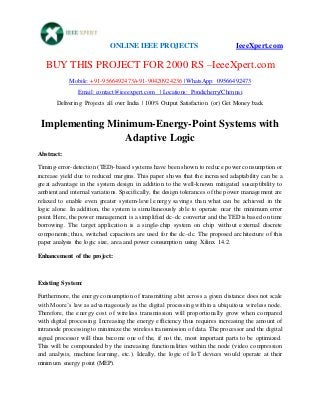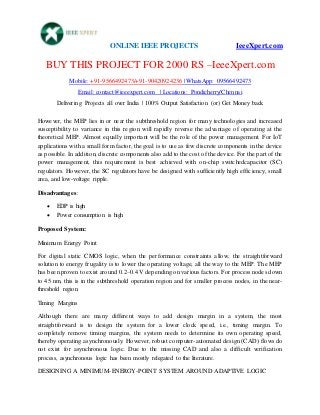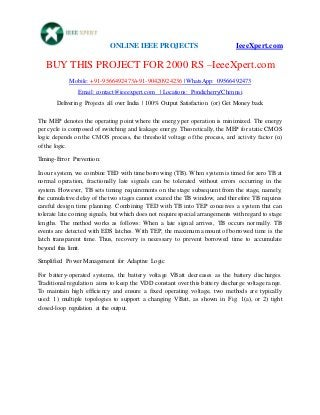Successfully reported this slideshow.

# Implementing minimum energy-point systems with adaptive logic×

# Implementing minimum energy-point systems with adaptive logic

Implementing minimum energy-point systems with adaptive logic Implementing minimum energy-point systems with adaptive logic Implementing minimum energy-point systems with adaptive logic Implementing minimum energy-point systems with adaptive logic Implementing minimum energy-point systems with adaptive logic

Implementing minimum energy-point systems with adaptive logic Implementing minimum energy-point systems with adaptive logic Implementing minimum energy-point systems with adaptive logic Implementing minimum energy-point systems with adaptive logic Implementing minimum energy-point systems with adaptive logic